# Constant-depth circuits for dynamic simulations of materials on quantum computers

## Abstract

Dynamic simulation of materials is a promising application for near-term quantum computers. Current algorithms for Hamiltonian simulation, however, produce circuits that grow in depth with increasing simulation time, limiting feasible simulations to short-time dynamics. Here, we present a method for generating circuits that are constant in depth with increasing simulation time for a specific subset of one-dimensional (1D) materials Hamiltonians, thereby enabling simulations out to arbitrarily long times. Furthermore, by removing the effective limit on the number of feasibly simulatable time-steps, the constant-depth circuits enable Trotter error to be made negligibly small by allowing simulations to be broken into arbitrarily many time-steps. For an N-spin system, the constant-depth circuit contains only $$\mathcal {O}(N^{2})$$ CNOT gates. Such compact circuits enable us to successfully execute long-time dynamic simulation of ubiquitous models, such as the transverse field Ising and XY models, on current quantum hardware for systems of up to 5 qubits without the need for complex error mitigation techniques. Aside from enabling long-time dynamic simulations with minimal Trotter error for a specific subset of 1D Hamiltonians, our constant-depth circuits can advance materials simulations on quantum computers more broadly in a number of indirect ways.

## Introduction

Quantum computers are intrinsically fit for efficiently simulating quantum systems (Feynman 1982; Lloyd 1996; Abrams and Lloyd 1997; Zalka 1998), making the simulation of quantum materials a leading “killer application” for this novel technology. Near-term quantum computers, also known as noisy intermediate-scale quantum (NISQ) computers, suffer from short qubit decoherence times and high gate error rates, making it difficult to achieve high-fidelity results with large quantum circuits (Preskill 2018). Thus, one of the major challenges with performing simulations on current quantum hardware is keeping the circuits small enough such that their results remain distinguishable from random noise.

This is particularly challenging for dynamic simulations, which require the execution of one circuit per time-step, where each circuit implements the time-evolution operator from the initial time to the given time-step (Bassman Oftelie et al. 2021). Current algorithms for dynamic materials simulations produce quantum circuits whose depths grow with increasing time-step count (Wiebe et al. 2011; Childs et al. 2018). Thus, an essential part of the workflow is quantum circuit optimization, which aims to minimize the depth of the circuits. Already, a great deal of research has focused on general circuit optimization (i.e. minimization) (Möttönen et al. 2004; De Vos and De Baerdemacker 2016; Iten et al. 2016; Martinez et al. 2016; Khatri et al. 2019; Murali et al. 2019; Younis et al. 2021; Cincio et al. 2020), which is an NP-hard problem (Botea et al. 2018; Herr et al. 2017). More recently, domain-specific circuit optimizers, which focus on optimizing certain types of circuits for specific applications, have been suggested (Bassman Oftelie et al. 2020) as a method to reduce to complexity of this optimization problem.

According to the “no-fast-forwarding theorem”, simulating the dynamics of a system under a generic Hamiltonian H for a time t requires Ω(t) gates (Berry et al. 2007; Childs and Kothari 2010), implying that circuit depths grow at least linearly with the number of time-steps. It has been shown, however, that quadratic Hamiltonians can be fast-forwarded, meaning the evolution of the systems under such Hamiltonians can be simulated with circuits whose depths do not grow significantly with the simulation time (Atia and Aharonov 2017; Gu et al. 2021). A recent work took advantage of this to variationally compile approximate circuits with a hybrid classical-quantum algorithm for fast-forwarded simulations (Cîrstoiu et al. 2020). The circuits, however, are approximate, with error that grows with increasing fast-forwarding time.

Here, we present an algorithm for generating quantum circuits that are constant in depth with increasing time-step count for simulations of materials governed by a specific set of models derived from the one-dimensional (1D) Heisenberg Hamiltonian, which we denote as $$\mathcal {H_{CD}}$$ and define in Theoretical background section. This set $$\mathcal {H_{CD}}$$, whose most prominent models include the transverse field Ising model (TFIM) and the (transverse field) XY model, is characterized by Hamiltonians that can be mapped to free fermionic models. While such models are known to be classically simulatable with polynomial resources (Valiant 2002; Terhal and DiVincenzo 2002), our constant-depth circuits can nonetheless help advance current research into materials simulations on near-term quantum computers. This is illustrated by the ubiquity of these models in current research on near-term quantum computers (Zhukov et al. 2018; Lamm and Lawrence 2018; Gustafson et al. 2019; Zhu et al. 2020; Bassman Oftelie et al. 2020; Yeter-Aydeniz et al. 2021; Bassman Oftelie et al. 2021; Sun et al. 2021; Neill et al. 2021). Indeed, systems like the TFIM are quintessential in the study of quantum phase transitions (Suzuki et al. 2012; Gómez-Ruiz et al. 2016; Yang et al. 2019), ergodicity (Cheraghi and Mahdavifar 2020), critical behavior (Granato 1992), as well as myriad condensed matter systems, such as ferroelectrics (Blinc et al. 1979) and magnetic spin glasses (Wu et al. 1991). When these models are made time-dependent, non-equilibrium effects such as dynamic phase transitions and quantum hysteresis can be studied (Tomé and de Oliveira 1990; Acharyya and Chakrabarti 1995; Acharyya 1998; Sides et al. 1998).

The constant-depth circuits for simulating the dynamics of models in $$\mathcal {H_{CD}}$$ are comprised of two-qubit gates, known as matchgates (Valiant 2002). While generic two-qubit gates decompose into native-gate circuits with at most three CNOT gates (Vidal and Dawson 2004), the matchgates in our constant-depth circuits only require two CNOT gates in their decomposition. This special property allows us to introduce a set of conjectured matchgate identities, which enable the downfolding of our circuits for dynamic simulations into constant-depth for any number of time-steps.

The circuits have a fixed structure, with only the single-qubit rotation angles changing with the addition of more time-steps. The structure has a regular pattern which can be easily extrapolated to build circuits for any system size; for an N-spin system, the circuit structure contains only N(N−1) CNOT gates. Furthermore, the circuits are exact up to Trotter error, which we argue, can be practically eliminated. This is because Trotter error scales with the size of the simulation time-step, and the constant-depth nature of the circuits allows for a simulation to be feasibly broken into arbitrarily many (i.e., arbitrarily small) time-steps. For a given system size, if the constant-depth circuit is small enough to achieve high-fidelity results on a NISQ computer, the dynamics of that system can be successfully simulated out to arbitrarily long times and with arbitarily small Trotter error.

## Theoretical background

The quantum circuits for dynamic simulations of quantum materials must implement the time-evolution operator between the initial time (which we set to 0) and some final time t, given by

$$U(0,t)\equiv U(t)=\mathcal{T} \exp(-i\int_{0}^{t}H(t)dt)$$
(1)

where $$\mathcal {T}$$ indicates a time-ordered exponential and H(t) is the time-dependent Hamiltonian of the material. In general, this operator is challenging to compute exactly due to the time-dependence of the Hamiltonian and the exponentiation of the Hamiltonian. Typically, the Trotter decomposition (Trotter 1959) is used to approximately construct U(t). With this method, the time-dependent Hamiltonian H(t) is first approximated as a piece-wise constant function by discretizing time into small time-steps over which H(t) is constant (Poulin et al. 2011). Next, the Hamiltonian at each time-step is split into components that are each individually easy to diagonalize, which enables Trotter decomposition to be performed at each time-step. In this way, the time-evolution operator is approximated as:

$$U(n\Delta t) = \prod_{\tau=1}^{n} \prod_{l} e^{-iH_{l}(t_{\tau})\Delta t} + \mathcal{O}(\Delta t)$$
(2)

where τ multiplies over the number of discretized time-steps Δt and l multiplies over the components into with H(t) was divided. We note that other techniques for approximating the unitary operator exist (Childs and Wiebe 2012; Chen et al. 2021), but are rarely used in practice at present as the circuits they produce are far too large for current hardware.

The error generated from the Trotter decomposition, known as Trotter error, can be a significant source of error, scaling with the size of the simulation time-step Δt. Dynamic simulations based on Trotter decomposition must therefore strike a balance when selecting the size of Δt. This is because standard algorithms for such simulations produce circuits which grow in depth with increasing numbers of time-steps, which in turn limits the number of time-steps that are feasible to simulate to just a handful (Smith et al. 2019). While decreasing Δt will lower Trotter error, making Δt too small will not allow for a long enough total simulation time, since the number of time-steps is limited. Our constant-depth circuits, however, remove the limitation on the number of time-steps that can be feasibly simulated, since the circuits do not get deeper with higher time-step count. This allows for the time-step Δt to be made arbitrarily small, which in turn allows one to decrease the Trotter error to negligible values. Such practical elimination of Trotter error with constant-depth circuits can enable far more accurate simulation results for long-time dynamic simulations.

The constant-depth circuits we introduce here simulate the dynamical evolution of a quantum material whose Hamiltonian is a simplified version of the 1D Heisenberg model, as explained below. The Heisenberg Hamiltonian is defined as

$$H(t) = -\sum_{\alpha}\{J_{\alpha}\sum_{i=1}^{N-1} \sigma_{i}^{\alpha}\sigma_{i+1}^{\alpha}\} - h_{\beta}(t) \sum_{i=1}^{N} \sigma_{i}^{\beta}$$
(3)

where α sums over {x,y,z}, the coupling parameters Jα denote the exchange interaction between nearest-neighbor spins along the α-direction, $$\sigma _{i}^{\alpha }$$ is the α-Pauli matrix acting on qubit i, and hβ(t) is the time-dependent amplitude of an external magnetic field along the β-direction, where β{x,y,z}. This Hamiltonian is thus defined by the set of its parameters {Jx,Jy,Jz,hβ(t)}. We denote the set of all parameter sets as $$\mathcal {H}$$. The full Heisenberg model is obtained when all parameters in the set are non-zero, however a number of ubiquitous models can be derived by setting various parameters to zero.

Table 1 shows all subsets $$\mathcal {H_{CD}}$$ of $$\mathcal {H}$$ for which we find that our constant-depth circuits work. The rows of the table denote either the direction of the external magnetic field hβ or a lack of field, while the columns label which of the coupling parameters are non-zero. The first three columns denote parameter sets where one coupling term is non-zero, the next three columns denote sets where two coupling terms are non-zero, while the final column denotes the sets where all three coupling parameters are non-zero. An × appears in table entries for parameter sets that define Hamiltonians in $$\mathcal {H_{CD}}$$, which can be simulated with our constant-depth circuits. Note that Jx·Jy·Jz=0 is a necessary but not sufficient condition for constant-depth. We remark that all Hamiltonians in $$\mathcal {H_{CD}}$$ can be mapped to free fermionic models.

As all $$\mathcal {H_{CD}}$$ Hamiltonians of Table 1 are quadratic, it is possible to fast-forward simulations under their time-evolution (Atia and Aharonov 2017; Gu et al. 2021). In Demonstration of constant-depth circuits section, we demonstrate simulations with our constant-depth circuits for two important models in $$\mathcal {H_{CD}}$$: (i) the XY model, where Jz=0 and hβ=0, and (ii) the TFIM, where Jy=Jz=0. Dynamics of these models have recently been simulated on quantum computers, but lack of constant-depth circuits limited the number of time-steps that could be successfully simulated (Smith et al. 2019).

## Construction of constant-depth circuits

To arrive at the circuit structure for the constant-depth circuits, we begin by laying down the gates that implement evolution of the system by one time-step, U(Δt). Due to the quadratic nature of $$\mathcal {H_{CD}}$$ Hamiltonians, which only contain coupling interactions between nearest neighbor spins, the circuit for evolution of one time-step can be constructed by a set of two-qubit gates which act on each of the pairs of nearest neighbor qubits. For example, for six qubits, this circuit is given by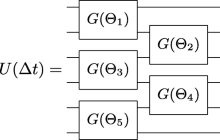(4)

where each gate labeled G(Θi) is a two-qubit gate defined by some set of parameters Θi. For ease of notation, the parameter set Θi is dropped in subsequent labeling of these gates, which will simply be labeled with a G. However, it must be emphasized that each two-qubit gate has its own unique parameter set. Each additional time-step requires one additional repetition of the circuit for one time-step. In this manner, it is possible to construct circuits for dynamic simulations that grow with increasing numbers of time-steps. We refer to these circuits as “growing depth circuits” for dynamic simulations. The growing depth circuit for n time-steps for six qubits is thus given by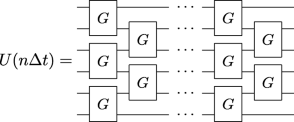(5)

where there are 2n columns of G gates for n time-steps. We now show that it is possible to reduce the growing depth circuits for higher numbers of time-steps down to a constant-depth circuit which is comprised of N columns of G gates for an N-spin system, where each column alternates placing the top of the first G gate on the first or second qubit. The ability to “downfold” longer circuits into constant-depth circuits is derived from special properties of these G gates.

In fact, the G gates belong to a special group of two-qubit gates known as matchgates (Valiant 2002).

### Definition 1

Let the matrices A and B be in SU(2)

$$\begin{array}{*{20}l} A &= \left[\begin{array}{cc} p & q \\ r & s \end{array}\right], & B &= \left[\begin{array}{cc} w & x \\ y & z \end{array}\right], \end{array}$$
(6)

with det(A)= det(B). Then the two-qubit matchgate G(A,B) is defined as follows

$$G(A,B) = \left[\begin{array}{cccc} p & & & q \\ & w & x \\ & y & z \\ r & & & s \end{array}\right].$$
(7)

Matchgates have the important property that the product of two matchgates is again a matchgate and this will be a key feature to arrive at constant-depth circuits.

### Lemma 1

Let G(A1,B1) and G(A2,B2) be matchgates, then the matrix

$$G(A_{3},B_{3}) = G(A_{1},B_{1}) \, G(A_{2},B_{2}),$$
(8)

is again a matchgate with A3=A1A2 and B3=B1B2.

### Proof

The proof directly follows from carrying out the matrix-matrix multiplication. □

A graphical representation of Lemma 1 is given by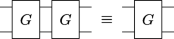(9)

The decomposition of a general matchgate into a native-gate circuit, which can be executed on NISQ devices, requires three CNOT gates (Vidal and Dawson 2004). However, all the matchgates for $$\mathcal {H_{CD}}$$ have a special structure which allows them to be decomposed into native-gate circuits with only two CNOT gates. In $$\mathcal {H_{CD}}$$ cases with an external magnetic field along the x- or y-directions, the gates G in (4) do not have the matchgate structure but are spectrally equivalent with a matchgate and are in fact matchgates up to some π/2 rotations. Matchgates and their corresponding decomposition into native-gate circuits with two-CNOTs are given for all Hamiltonians in $$\mathcal {H_{CD}}$$ in Appendix A.

The ability to contract the circuits to constant depth relies on an identity that we conjecture for these special $$\mathcal {H_{CD}}$$ matchgates.

### Conjecture 1

Let G1,G2,G3 be matchgates of a certain type in $$\mathcal {H_{CD}}$$, then there exist three corresponding matchgates G4,G5,G6 of the same type so that

$$(G_{1} \otimes I)(I \otimes G_{2})(G_{3} \otimes I) = (I \otimes G_{4})(G_{4} \otimes I)(I \otimes G_{6}).$$
(10)

A graphical representation of Conjecture 1 is given by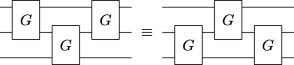(11)

Using numerical optimization to identify circuit parameters on either side of the equality, we empirically find this conjecture to be true for all trials where the circuits are comprised of $$\mathcal {H_{CD}}$$ matchgates. It has, however, proven challenging to analytically compute the parameters of the circuit on the right-hand side given the parameterized circuit on the left-hand side and vice versa. As a result, compilation of our constant-depth circuits requires numerical optimization to obtain circuit parameters. We emphasize that the equivalence (11) only holds for $$\mathcal {H_{CD}}$$ matchgates, whereas the equivalence (9) holds for all matchgates.

Based on Eqs. 9 and 11 we can derive identities for higher numbers of qubits, where a set of N columns of matchgates across N qubits can be replaced by its mirror image, albeit with altered parameter sets for all the constituent matchgates. A demonstration of deriving the identity for 4-qubits is shown in Appendix B. We refer to these identities as the matchgate mirroring identities. These conjectured identities are depicted in Fig. 1 for four and five qubits. Note that for even numbers of qubits the mirroring is about a vertical axis (Fig. 1a), while for odd numbers of qubits the mirroring is about a horizontal axis (Fig. 1b). We emphasize that the matchgate parameters are different on either side of the equality signs.

To understand how these mirroring identities allow for the construction of constant depth circuits, we notice that for an N-qubit growing depth circuit (e.g., Eq. 5) we can apply the matchgate mirroring identity to the last N columns of matchgates in the circuit. We note that applying this identity will change the parameters defining each matchgate within the mirroring group. Application of this identity will result in pairs of adjacent matchgates on the same qubit pairs that can be combined into one matchgate, thus reducing the number of columns of matchgates in the circuit by one. This can be repeated until only N columns of matchgates in the circuit remain. This process is demonstrated for six qubits in Fig. 2. Figure 2a shows the growing depth circuit for six qubits simulating n time-steps with the last six columns of matchgates in the circuit highlighted with an outline. Figure 2b shows one application of the matchgate mirroring identity for six qubits to these last six columns of matchgates. Note how after applying the identity, two pairs of matchgates emerge adjacent to one another on the same pair of qubits, highlighted with an outline. These pairs can each be merged into one matchgate with new parameters, thus reducing the number of columns of matchgates in the circuit by one. This process is repeated until only six columns of matchgates remain, as shown in Fig. 2c.

The downfolding approach presented in Fig. 2 shows how to methodically obtain constant-depth circuits for each time-step in the dynamic simulation. In practice, however, we directly use numerical optimization to find the parameters for the constant-depth circuit of Fig. 2c. We begin by computing the operator in Eq. 2 (either by exact diagonalization or Trotter decomposition), which defines our target matrix (i.e., the matrix our circuit aims to carry out). Given a system size, we then construct the constant-depth circuit structure, which has N columns of matchgates for an N-qubit system. Next, we compute the matrix equivalent of the circuit, which will be compared to our target matrix. Using numerical optimization, we then solve for the parameters of the circuit that minimize the distance between the circuit matrix and the target matrix.

The number of circuit parameters grows quadratically with system size. This makes scaling to larger system sizes challenging as the circuit optimization for each time-step will take longer to compute. This could be ameliorated by finding a way to map the coefficients of the Hamiltonian directly to the rotation angles in the constant-depth circuit, whether through analytical techniques or machine learning methods. This would enable one to skip computation of the time-evolution operator and numerical optimization altogether. It should be noted, however, that the inability to remove this classical optimization step may not completely inhibit this method because the constant-depth circuit generation is embarrassingly parallel. In other words, the circuits for each time-step may all be computed in parallel, as numerical optimization for one circuit does not depend on information from any other circuit. In this way, the numerical optimization of circuits for all time-steps for large system simulations could be executed simultaneously on a classical supercomputer, which are regularly used for similar computations.

The circuit volume of the constant-depth circuits grows quadratically with system size N, while the depth grows only linearly with N. We emphasize, however, that unlike previous circuit generation techniques, our circuits do not grow in size with increasing numbers of time-steps, but rather remain fixed for a given system size N. This remarkable feature is what enables simulation out to arbitrarily large numbers of time-steps and thus permits long-time dynamic simulations. Most other methods for circuit generation will produce circuits that grow linearly with increasing numbers of time-steps (Wiebe et al. 2011; Smith et al. 2019). This prohibits dynamic simulations beyond a certain number of time-steps due to the quantum computer encountering circuits that are too large, and thus accumulate too much error due to gate errors and qubit decoherence.

## Demonstration of constant-depth circuits

To demonstrate the power of our constant-depth circuits, we simulate quantum quenches of 3-, 4-, and 5-spin systems defined by the TFIM and XY model on the IBM quantum processor “ibmq_athens”. A quantum quench is simulated by initializing the system in the ground state of an initial Hamiltonian, Hi, and then evolving the system through time under a final Hamiltonian, Hf. Quenches can simulate a sudden change in a system’s environment and provide insights into the non-equilibrium dynamics of various quantum materials.

The TFIM is obtained by setting Jy=Jz=0 and β=z in the Hamiltonian in Eq. 3. To perform a quench with the TFIM, we assume the external magnetic field is initially turned off, and the qubits are initialized in the ground state of an initial Hamiltonian $$H_{i}(t < 0) = \sum _{i} -J_{x}\ \sigma _{i}^{x}\sigma _{i+1}^{x}$$, which is a ferromagnetic state oriented along the x-axis. At time t=0, a time-dependent magnetic field is instantaneously turned on, and the system evolves under the final Hamiltonian $$H_{i}(t \ge 0) = -\sum _{i} \{J_{x}\sigma _{i}^{x}\sigma _{i+1}^{x} + h_{z}(t)\sigma _{i}^{z}\}$$, which represents the TFIM. We use parameters from Ref Bassman Oftelie et al. (2020), setting Jx=11.83898 meV and hz(t)=2Jx cos(ωt) with ω=0.0048 fs−1, which simulates a simplified model of a Re-doped mono-layer of MoSe2 under laser excitation. A time-step of 3 fs is used in the simulations. Our observable of interest is the average magnetization of the system along the x-axis, given by $$m_{x}(t) = \frac {1}{N}\sum _{i} \langle \sigma _{i}^{x}(t)\rangle$$.

The XY model is obtained by setting Jz=hβ=0 in the Hamiltonian in Eq. 3. To perform a quench with the XY model, we initially let Jz and approximate the initial Hamiltonian $$H_{i}(t<0) = C \sum _{i}\sigma _{i}^{z}\sigma _{i+1}^{z}$$, where C is an arbitrarily large constant. The ground state of this Hamiltonian is the Néel state, defined as |ψ0〉=|〉. At time t=0, we instantaneously set Jz=0, and let Jx=Jy=−1.0 eV, giving a final Hamiltonian of $$H_{f}(t \geq 0) = \sum _{i}\{\sigma _{i}^{x}\sigma _{i+1}^{x} + \sigma _{i}^{y}\sigma _{i+1}^{y}\}$$, which represents the XY model. A time-step of 0.025 fs is used in the simulations. Our observable of interest is the staggered magnetization of the system, which is related to the antiferromagnetic order parameter and given by $$m_{s}(t) = \frac {1}{N}\sum _{i} (-1)^{i} \langle \sigma _{i}^{z}(t)\rangle$$.

To generate the constant-depth circuits for our simulations, we rely on circuit optimization software provided by the circuit synthesis toolkit BQSKit (Berkeley Quantum Synthesis Toolkit 2021). This suite of software provides several packages which can be used to generate the constant-depth circuits. The user must provide the matrix representation for the time-evolution operator to be implemented along with the parameter-dependent constant-depth circuit structure. The circuit synthesis software then proceeds to use numerical optimization to find the optimal parameters for the circuit. Tutorials including the full code for generating our constant-depth circuits using the BQSKit toolkit are included in the Supplemental Material (Bassman Oftelie et al. 2021).

Figure 3 shows the simulation results for quenches of the TFIM (top row) and XY model (bottom row) for various system sizes performed on a real quantum processor. The magnetization for each time-step was average over 8192 shots. In the first three columns, the results from our constant-depth circuits (red) and growing depth circuits (green) are compared to the expected results computed with a noise-free quantum computer simulator (blue). We note that time-step size was chosen sufficiently small such that the noise-free simulator results are in complete agreement with numerically exact results computed with exact diagonalization, and thus serve as our “ground truth”. The growing depth circuits were generated from standard Trotter decomposition and optimized with the IBM native compiler. While some new techniques have been developed for making shorter circuits based on Trotterization (Childs et al. 2018; Campbell 2019; Tran et al. 2020; Kivlichan et al. 2020; Childs et al. 2021), they nonetheless still grow in size with increasing time-step count, and thus will still generate results indistinguishable from random noise beyond a certain time-step. For this reason, the growing depth circuits produce qualitatively consistent results for the first few time-steps, but thereafter the circuits are too large, accumulating too much error, to produce high-fidelity results. A recent benchmark study of dynamic simulations of similar systems on quantum computers found analogous behavior, with high-fidelity results limited to only a handful of time-steps (Smith et al. 2019). In contrast, the results from our constant-depth circuits remain accurate for all time-step counts, and in principle, will remain so out to arbitrarily many time-steps. These results thus show the power of constant-depth circuits to enable long-time dynamic simulations.

Figure 3d and h compare the number of CNOT gates for each time-step in the constant-depth (red) and growing depth (green) circuits for 3- (dotted line), 4- (dashed lined), and 5-spin (solid line) systems. Clearly the number of CNOT gates remains the same for all time-steps for our constant-depth circuits, but the number grows linearly with increasing numbers of time-steps for the growing depth circuits. Notice how the number of CNOT gates per time-step for the XY model circuits (3h) are approximately double the number for the TFIM circuits (3d), while our constant-depth circuits have the same CNOT count for both models.

## Discussion and outlook

Standard Hamiltonian simulation algorithms produce circuits that grow in depth with increasing time-step count, which limits the number of time-steps that are feasible to simulate on near-term quantum devices. The constant-depth circuits we have presented remove this limit when simulating a specific set of 1D Hamiltonian models, namely those which can be mapped to free fermionic models, including the TFIM and XY model. Simulations of these systems, therefore, can be broken into arbitrarily many time-steps, which allows for Trotter error to be made negligibly small and enables long-time dynamics to be more feasibly simulated on near-term quantum devices. While simulations of free-fermionic systems are known to be classically simulatable with resources that scale polynomially with system size (Valiant 2002; Terhal and DiVincenzo 2002), there are numerous ways in which the constant-depth can indirectly contribute to progressing dynamic simulations on materials on near-term quantum computers. For example, our constant-depth circuits can enable preparation of non-trivial ground states of $$\mathcal {H_{CD}}$$ models through adiabatic state preparation (ASP). Since our compressed circuits allow for arbitrarily many time-steps in a simulation, this allows arbitrarily slow (i.e., adiabatic) evolution under a time-dependent Hamiltonian, which is the basis for ASP (Aspuru-Guzik et al. 2005; Barends et al. 2016). In this way, our circuits might be used as an initial state preparation sub-circuit within a larger simulation circuit. Indeed, our compressed circuits may serve as sub-circuits in any larger circuit that contains a component with time-evolution under one of the $$\mathcal {H_{CD}}$$ Hamiltonians. Another example can be seen in Ref. Bassman Oftelie et al. (2021), where a finite temperature state was prepared in an initial part of a simulation circuit and our compressed circuit was appended to time evolve the initial thermal state.

Other areas of utility for our compressed circuits include the benchmarking of new error mitigation or noise extrapolation techniques (Gustafson et al. 2019; Li and Benjamin 2017), as well as the benchmarking of the performance of quantum hardware in general. For these endeavours our constant-depth circuits can prove highly useful as they are feasible to run on near-term hardware and are classically efficiently simulatable, which allows for the calculation of a ground truth for use in the benchmarking. Finally, future work might also explore if and how our constant-depth circuits can be adapted for approximations of various extensions of the Hamiltonians in $$\mathcal {H_{CD}}$$, including two-dimensional models, models with next-nearest neighbor or even longer-range interactions, or full Heisenberg interactions (i.e., coupling interactions along three axes). Indeed, matchgates have previously been studied for various two-dimensional qubit topologies and for longer-range interactions (Brod and Galvão 2012; Brod and Childs 2014). Paired with incremental improvements in quantum hardware, the ability to extend our constant-depth circuits to more complex systems could pave the way to new discoveries in the behavior of quantum materials by enabling long-time dynamic simulations on quantum computers of systems relevant to scientific and technological problems.

## Appendix A: quantum circuits for $$\mathcal {H}_{\mathcal {CD}}$$ matchgates

Here, we describe the matchgates used for the various Hamiltonians in $$\mathcal {H_{CD}}$$ in terms of their matrix representation, as well as their quantum circuit representation. In the following, we use θi to represent the free parameters of the circuit that must be set by the optimizer for a particular Hamiltonian and time-step. Rx(θ),Ry(θ), and Rz(θ) are rotation gates, which rotate the qubit around the x-, y-, z-axis, respectively, by an angle θ. Elements of the matchgate matrix representations in plain text are real numbers, while elements in colored, boldface text are complex numbers. A bar over a complex element denotes its complex conjugate.

### Hamiltonian parameter subsets with: JxJy=JxJz=JyJz=0 and hβ=0

#### Matchgates used in constant-depth circuits for simulating $$H = j_{x} \sum _{i} \sigma _{i}^{x} \sigma _{i+1}^{x}$$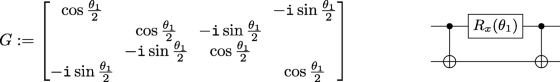(12)

#### Matchgates used in constant-depth circuits for simulating $$H = j_{y} \sum _{i} \sigma _{i}^{y} \sigma _{i+1}^{y}$$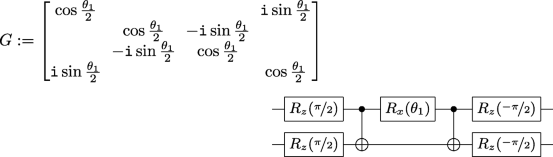(13)

#### Matchgates used in constant-depth circuits for simulating $$H = j_{z} \sum _{i} \sigma _{i}^{z} \sigma _{i+1}^{z}$$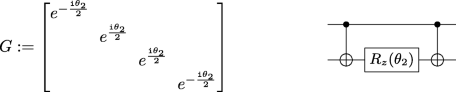(14)

### Hamiltonian parameter subsets with: JxJy=JxJz=JyJz=0 and hβ≠0

#### G gates used in constant-depth circuits for simulating $$H = j_{x} \sum _{i} \sigma _{i}^{x} \sigma _{i+1}^{x} + h_{x}\sum _{i} \sigma _{i}^{x}$$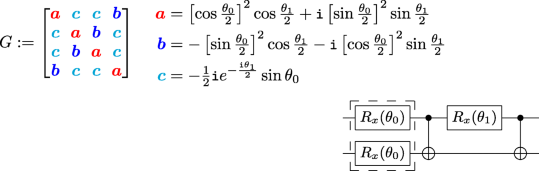(15)

#### G gates used in constant-depth circuits for simulating $$H = j_{y} \sum _{i} \sigma _{i}^{y} \sigma _{i+1}^{y} + h_{y}\sum _{i} \sigma _{i}^{y}$$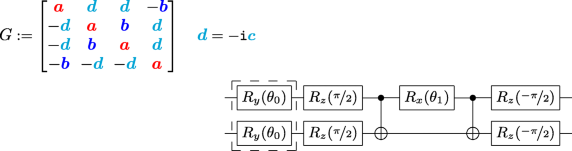(16)

#### Matchgates used in constant-depth circuits for simulating $$H = j_{z} \sum _{i} \sigma _{i}^{z} \sigma _{i+1}^{z} + h_{z}\sum _{i} \sigma _{i}^{z}$$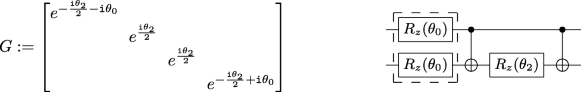(17)

### Hamiltonian parameter subsets with: JxJyJz=0 and hβ=0

#### Matchgates used in constant-depth circuits for simulating $$H = \sum _{i} j_{x}\sigma _{i}^{x} \sigma _{i+1}^{x} + j_{y}\sigma _{i}^{y} \sigma _{i+1}^{y}$$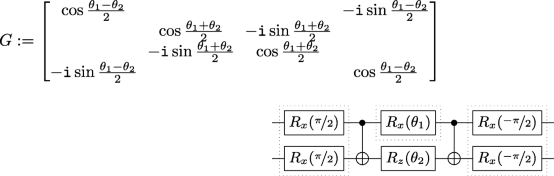(18)

#### Matchgates used in constant-depth circuits for simulating $$H = \sum _{i} j_{x}\sigma _{i}^{x} \sigma _{i+1}^{x} + j_{z}\sigma _{i}^{z} \sigma _{i+1}^{z}$$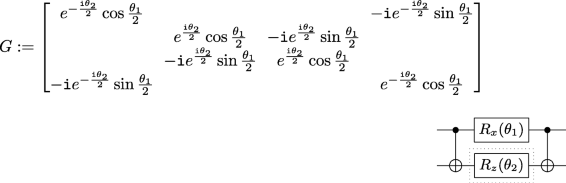(19)

#### Matchgates used in constant-depth circuits for simulating $$H = \sum _{i} j_{y}\sigma _{i}^{y} \sigma _{i+1}^{y} + j_{z}\sigma _{i}^{z} \sigma _{i+1}^{z}$$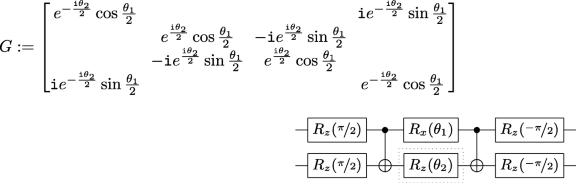(20)

### Hamiltonian parameter subsets with: JxJyJz=0 and hβ≠0

#### Matchgates used in constant-depth circuits for simulating $$H = j_{x}\sum _{i} \sigma _{i}^{x} \sigma _{i+1}^{x} + h_{z}\sum _{i} \sigma _{i}^{z}$$ or $$H = j_{y}\sum _{i} \sigma _{i}^{y} \sigma _{i+1}^{y} + h_{z}\sum _{i} \sigma _{i}^{z}$$ or $$H = \sum _{i} \{J_{x}\sigma _{i}^{x} \sigma _{i+1}^{x} + j_{y}\sigma _{i}^{y} \sigma _{i+1}^{y}\} + h_{z}\sum _{i} \sigma _{i}^{z}$$

$${}G := \left[\begin{array}{cccc} e^{-\texttt{i}(\theta_{0} + \theta_3)}\cos\frac{\theta_{1} - \theta_{2}}{2} & & & -\texttt{i} e^{\texttt{i}(\theta_{0} - \theta_3)}\sin\frac{\theta_{1} - \theta_{2}}{2} \\ & \cos\frac{\theta_{1} + \theta_{2}}{2} & -\texttt{i}\sin\frac{\theta_{1} + \theta_{2}}{2} \\ & -\texttt{i}\sin\frac{\theta_{1} + \theta_{2}}{2} & \cos\frac{\theta_{1} + \theta_{2}}{2} \\ -\texttt{i} e^{-\texttt{i}(\theta_{0} - \theta_3)}\sin\frac{\theta_{1} - \theta_{2}}{2} & & & e^{\texttt{i}(\theta_{0} + \theta_3)}\cos\frac{\theta_{1} - \theta_{2}}{2} \\ \end{array}\right]$$
(21a)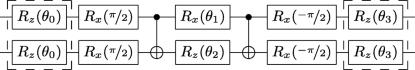(21b)

#### G gates used in constant-depth circuits for simulating $$H = j_{x}\sum _{i} \sigma _{i}^{x} \sigma _{i+1}^{x} + h_{y}\sum _{i} \sigma _{i}^{y}$$ or $$H = j_{z}\sum _{i} \sigma _{i}^{z} \sigma _{i+1}^{z} + h_{y}\sum _{i} \sigma _{i}^{y}$$ or $$H = \sum _{i} \{J_{x}\sigma _{i}^{x} \sigma _{i+1}^{x} + j_{z}\sigma _{i}^{z} \sigma _{i+1}^{z}\} + h_{y}\sum _{i} \sigma _{i}^{y}$$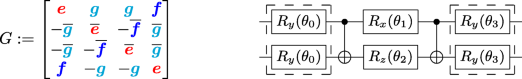(22a)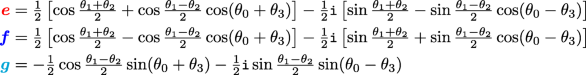(22b)

#### G gates used in constant-depth circuits for simulating $$H = j_{y}\sum _{i} \sigma _{i}^{y} \sigma _{i+1}^{y} + h_{x}\sum _{i} \sigma _{i}^{x}$$ or $$H = j_{z}\sum _{i} \sigma _{i}^{z} \sigma _{i+1}^{z} + h_{x}\sum _{i} \sigma _{i}^{x}$$ or $$H = \sum _{i} \{J_{y}\sigma _{i}^{y} \sigma _{i+1}^{y} + j_{z}\sigma _{i}^{z} \sigma _{i+1}^{z}\} + h_{x}\sum _{i} \sigma _{i}^{x}$$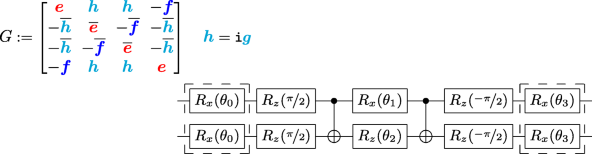(23)

## Appendix B: Proof of Fig. 1a

### Proof

Using (11) recursively, yields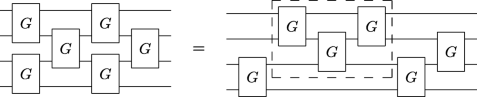(24)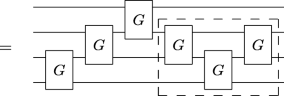(25)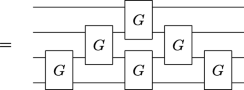(26)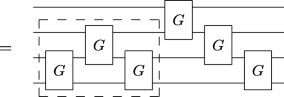(27)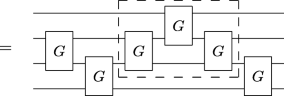(28)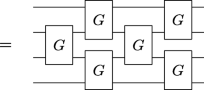(29)

## Availability of data and material

Code to generate our constant-depth circuits can be found in the following GitHub repository: https://github.com/lebassman/Constant_Depth_Circuits.

## Abbreviations

1D:

One-Dimensional

NISQ:

Noisy Intermediate-Scale Quantum

TFIM:

Transverse Field Ising Model

ASP:

## References

• D. S. Abrams, S. Lloyd, Simulation of many-body Fermi systems on a universal quantum computer. Phys. Rev. Lett.79(13), 2586–2589 (1997). https://doi.org/10.1103/PhysRevLett.79.2586.

• M. Acharyya, Nonequilibrium phase transition in the kinetic ising model: Is the transition point the maximum lossy point?Phys. Rev. E. 58(1), 179 (1998).

• M. Acharyya, B. K. Chakrabarti, Response of ising systems to oscillating and pulsed fields: Hysteresis, ac, and pulse susceptibility. Phys. Rev. B. 52(9), 6550 (1995).

• A. Aspuru-Guzik, A. D. Dutoi, P. J. Love, M. Head-Gordon, Simulated quantum computation of molecular energies. Science. 309(5741), 1704–1707 (2005).

• Y. Atia, D. Aharonov, Fast-forwarding of Hamiltonians and exponentially precise measurements. Nat. Commun.8(1), 1572 (2017). https://doi.org/10.1038/s41467-017-01637-7.

• R. Barends, A. Shabani, L. Lamata, J. Kelly, A. Mezzacapo, U. Las Heras, R. Babbush, A. G. Fowler, B. Campbell, Y. Chen, et al, Digitized adiabatic quantum computing with a superconducting circuit. Nature. 534(7606), 222–226 (2016).

• L. Bassman Oftelie, S. Gulania, C. Powers, R. Li, T. Linker, K. Liu, T. K. S. Kumar, R. K. Kalia, A. Nakano, P. Vashishta, Domain-specific compilers for dynamic simulations of quantum materials on quantum computers. Quantum Sci. Technol.6(1), 14007 (2020). https://doi.org/10.1088/2058-9565/abbea1.

• L. Bassman Oftelie, K. Klymko, N. M. Tubman, W. A. de Jong, Computing free energies with fluctuation relations on quantum computers (2021). https://arxiv.org/abs/2103.09846. Accessed 15 Dec 2021.

• L. Bassman Oftelie, K. Liu, A. Krishnamoorthy, T. Linker, Y. Geng, D. Shebib, S. Fukushima, F. Shimojo, R. K. Kalia, A. Nakano, et al, Towards simulation of the dynamics of materials on quantum computers. Phys. Rev. B. 101(18), 184305 (2020). https://doi.org/10.1103/PhysRevB.101.184305.

• L. Bassman Oftelie, M. Urbanek, M. Metcalf, J. Carter, A. F. Kemper, W. A. de Jong, Simulating Quantum Materials with Digital Quantum Computers. Quantum Sci. Technol.6:, 043002 (2021).

• L. Bassman Oftelie, R Van Beeumen, E Younis, E Smith, Constant Depth Circuits for Dynamic Simulations of Materials on Quantum Computers (2021). https://github.com/lebassman/Constant_Depth_Circuits. Accessed 15 Dec 2021.

• Berkeley Quantum Synthesis Toolkit (2021). https://bqskit.lbl.gov. Accessed 15 Dec 2021.

• D. W. Berry, G. Ahokas, R. Cleve, B. C. Sanders, Efficient quantum algorithms for simulating sparse Hamiltonians. Comm. Math. Phys.270(2), 359–371 (2007). https://doi.org/10.1007/s00220-006-0150-x.

• R. Blinc, B. žekš, J. Sampaio, A. Pires, F. S. Barreto, Ising model in a transverse tunneling field and proton-lattice interaction in H-bonded ferroelectrics. Phys. Rev. B. 20(5), 1991 (1979).

• A. Botea, A. Kishimoto, R. Marinescu, in Proceedings of the 11th International Symposium on Combinatorial Search (SoCS 2018). On the complexity of quantum circuit compilation (AAAI PressPalo Alto, 2018), pp. 138–142.

• D. J. Brod, A. M. Childs, The computational power of matchgates and the XY interaction on arbitrary graphs. Quantum Inf. Comput.14(11-12), 901–916 (2014). https://doi.org/10.26421/QIC14.11-12.

• D. J. Brod, E. F. Galvão, Geometries for universal quantum computation with matchgates. Phys. Rev. A. 86(5), 52307 (2012). https://doi.org/10.1103/PhysRevA.86.052307.

• E. Campbell, Random compiler for fast hamiltonian simulation. Phys. Rev. Lett.123(7), 070503 (2019).

• Y. -H. Chen, A. Kalev, I. Hen, A quantum algorithm for time-dependent hamiltonian simulation by permutation expansion (2021). https://arxiv.org/abs/2103.15334. Accessed 15 Dec 2021.

• H. Cheraghi, S. Mahdavifar, probing the possibilities of ergodicity in the 1d spin-1/2 xy chain with quench dynamics. Sci. Rep.10(1), 1–10 (2020).

• A. M. Childs, R. Kothari, Limitations on the simulation of non-sparse Hamiltonians. Quantum Inf. Comput.10(7-8), 669–684 (2010). https://doi.org/10.26421/QIC10.7-8-7.

• A. M. Childs, D. Maslov, Y. Nam, N. J. Ross, Y. Su, Toward the first quantum simulation with quantum speedup. Proc. Natl. Acad. Sci.115(38), 9456–9461 (2018).

• A. M. Childs, Y. Su, M. C. Tran, N. Wiebe, S. Zhu, Theory of trotter error with commutator scaling. Phys. Rev. X. 11(1), 011020 (2021).

• A. M. Childs, N. Wiebe, Hamiltonian simulation using linear combinations of unitary operations (2012). https://arxiv.org/abs/1202.5822. Accessed 15 Dec 21.

• L. Cincio, K. Rudinger, M. Sarovar, P. J. Coles, Machine learning of noise-resilient quantum circuits. PRX Quantum. 2:, 010324 (2020).

• C. Cîrstoiu, Z. Holmes, J. Iosue, L. Cincio, P. J. Coles, A. Sornborger, Variational fast forwarding for quantum simulation beyond the coherence time. NPJ Quantum Inf.6(1), 82 (2020). https://doi.org/10.1038/s41534-020-00302-0.

• A. De Vos, S. De Baerdemacker, Block-ZXZ synthesis of an arbitrary quantum circuit. Phys. Rev. A.94(5), 52317 (2016). https://doi.org/10.1103/PhysRevA.94.052317.

• R. P. Feynman, Simulating physics with computers. Int. J. Theor. Phys.21(6), 467–488 (1982). https://doi.org/10.1007/BF02650179.

• F. Gómez-Ruiz, J. Mendoza-Arenas, F. Rodríguez, C. Tejedor, L. Quiroga, Quantum phase transitions detected by a local probe using time correlations and violations of leggett-garg inequalities. Phys. Rev. B. 93(3), 035441 (2016).

• E. Granato, Critical behavior of a one-dimensional frustrated quantum xy model. Phys. Rev. B. 45(5), 2557 (1992).

• S. Gu, R. D. Somma, B. Şahinoğlu, Fast-forwarding quantum evolution (2021). https://arxiv.org/abs/2105.07304. Accessed 15 Dec 21.

• E. Gustafson, P. Dreher, Z. Hang, Y. Meurice, Benchmarking quantum computers for real-time evolution of a (1+1) field theory with error mitigation. arXiv preprint arXiv:1910.09478 (2019).

• E. Gustafson, Y. Meurice, J. Unmuth-Yockey, Quantum simulation of scattering in the quantum ising model. Phys. Rev. D. 99(9), 094503 (2019).

• D. Herr, F. Nori, S. J. Devitt, Optimization of lattice surgery is NP-hard. NPJ Quantum Inf.3(1), 35 (2017). https://doi.org/10.1038/s41534-017-0035-1.

• R. Iten, R. Colbeck, I. Kukuljan, J. Home, M. Christandl, Quantum circuits for isometries. Phys. Rev. A. 93(3), 032318 (2016). https://doi.org/10.1103/PhysRevA.93.032318.

• S. Khatri, R. LaRose, A. Poremba, L. Cincio, A. T. Sornborger, P. J. Coles, Quantum-assisted quantum compiling. Quantum. 3:, 140 (2019). https://doi.org/10.22331/q-2019-05-13-140.

• I. D. Kivlichan, C. Gidney, D. W. Berry, N. Wiebe, J. McClean, W. Sun, Z. Jiang, N. Rubin, A. Fowler, A Aspuru-Guzik, et al, Improved fault-tolerant quantum simulation of condensed-phase correlated electrons via trotterization. Quantum. 4:, 296 (2020).

• H. Lamm, S. Lawrence, Simulation of nonequilibrium dynamics on a quantum computer. Phys. Rev. Lett.121(17), 170501 (2018).

• Y. Li, S. C. Benjamin, Efficient variational quantum simulator incorporating active error minimization. Phys. Rev. X. 7(2), 021050 (2017).

• S. Lloyd, Universal quantum simulators. Science. 273(5278), 1073–1078 (1996). https://doi.org/10.1126/science.273.5278.1073.

• E. A. Martinez, T. Monz, D. Nigg, P. Schindler, R. Blatt, Compiling quantum algorithms for architectures with multi-qubit gates. New J. Phys.18(6), 063029 (2016). https://doi.org/10.1088/1367-2630/18/6/063029.

• M. Möttönen, J. J. Vartiainen, V. Bergholm, M. M. Salomaa, Quantum circuits for general multiqubit gates. Phys. Rev. Lett.93(13), 130502 (2004). https://doi.org/10.1103/PhysRevLett.93.130502.

• P. Murali, J. M. Baker, A. Javadi-Abhari, F. T. Chong, M. Martonosi, in Proceedings of the Twenty-Fourth International Conference on Architectural Support for Programming Languages and Operating Systems (ASPLOS ’19). Noise-Adaptive Compiler Mappings for Noisy Intermediate-Scale Quantum Computers (ACM, 2019), pp. 1015–1029. https://doi.org/10.1145/3297858.3304075.

• C. Neill, T. McCourt, X. Mi, Z. Jiang, M. Niu, W. Mruczkiewicz, I. Aleiner, F. Arute, K. Arya, J. Atalaya, et al, Accurately computing the electronic properties of a quantum ring. Nature. 594(7864), 508–512 (2021).

• D. Poulin, A. Qarry, R. Somma, F. Verstraete, Quantum simulation of time-dependent Hamiltonians and the convenient illusion of Hilbert space. Phys. Rev. Lett.106(17), 170501 (2011). https://doi.org/10.1103/PhysRevLett.106.170501.

• J. Preskill, Quantum Computing in the NISQ era and beyond. Quantum. 2:, 79 (2018). https://doi.org/10.22331/q-2018-08-06-79.

• S. Sides, P. Rikvold, M. Novotny, Kinetic ising model in an oscillating field: Finite-size scaling at the dynamic phase transition. Phys. Rev. Lett.81(4), 834 (1998).

• A. Smith, M. S. Kim, F. Pollmann, J. Knolle, Simulating quantum many-body dynamics on a current digital quantum computer. NPJ Quantum Inf.5(1), 106 (2019). https://doi.org/10.1038/s41534-019-0217-0.

• S. -N. Sun, M. Motta, R. N. Tazhigulov, A. T. Tan, G. K. -L. Chan, A. J. Minnich, Quantum computation of finite-temperature static and dynamical properties of spin systems using quantum imaginary time evolution. PRX Quantum. 2(1), 010317 (2021).

• S. Suzuki, J. Inoue, B. K. Chakrabarti, Quantum Ising Phases and Transitions in Transverse Ising Models (Springer Berlin Heidelberg, Germany, 2012). https://books.google.com/books?id=y1S5BQAAQBAJ.

• B. M. Terhal, D. P. DiVincenzo, Classical simulation of noninteracting-fermion quantum circuits. Phys. Rev. A. 65(3), 032325 (2002).

• T. Tomé, M. J. de Oliveira, Dynamic phase transition in the kinetic ising model under a time-dependent oscillating field. Phys. Rev. A. 41(8), 4251 (1990).

• M. C. Tran, S. -K. Chu, Y. Su, A. M. Childs, A. V. Gorshkov, Destructive error interference in product-formula lattice simulation. Phys. Rev. Lett.124(22), 220502 (2020).

• H. F. Trotter, On the product of semi-groups of operators. Proc. Amer. Math. Soc.10(4), 545–551 (1959). https://doi.org/10.1112/jlms/s1-38.1.66.

• L. G. Valiant, Quantum circuits that can be simulated classically in polynomial time. SIAM J. Comput.31(4), 1229–1254 (2002). https://doi.org/10.1137/S0097539700377025.

• G. Vidal, C. M. Dawson, Universal quantum circuit for two-qubit transformations with three controlled-NOT gates. Phys. Rev. A. 69(1), 10301 (2004). https://doi.org/10.1103/PhysRevA.69.010301.

• N. Wiebe, D. W. Berry, P. Høyer, B. C. Sanders, Simulating quantum dynamics on a quantum computer. J. Phys. A Math. Theor.44(44), 445308 (2011). https://doi.org/10.1088/1751-8113/44/44/445308.

• W. Wu, B. Ellman, T. Rosenbaum, G. Aeppli, D. Reich, From classical to quantum glass. Phys. Rev. Lett.67(15), 2076 (1991).

• X. Yang, G. Zhang, Z. Song, Phase transition in phase transition lines of quantum xy model. J. Phys. Condens. Matter. 31(24), 245401 (2019).

• K. Yeter-Aydeniz, G. Siopsis, R. C. Pooser, Scattering in the ising model with the quantum lanczos algorithm. New J. Phys.23(4), 043033 (2021). https://doi.org/10.1088/1367-2630/abe63d.

• E. Younis, K. Sen, K. Yelick, C. Iancu, in 2021 IEEE International Conference on Quantum Computing and Engineering (QCE). QFAST: Conflating Search and Numerical Optimization for Scalable Quantum Circuit Synthesis, (2021), pp. 232–243. https://doi.org/10.1109/QCE52317.2021.00041.

• C. Zalka, Simulating quantum systems on a quantum computer. Proc. R. Soc. A. 454(1969), 313–322 (1998). https://doi.org/10.1098/rspa.1998.0162.

• D. Zhu, S. Johri, N. M. Linke, K. Landsman, C. H. Alderete, N. H. Nguyen, A. Matsuura, T. Hsieh, C. Monroe, Generation of thermofield double states and critical ground states with a quantum computer. Proc. Natl. Acad. Sci.117(41), 25402–25406 (2020).

• A. Zhukov, S. Remizov, W. Pogosov, Y. E. Lozovik, Algorithmic simulation of far-from-equilibrium dynamics using quantum computer. Quantum Inf. Process.17(9), 223 (2018).

## Acknowledgements

We acknowledge the use of IBM Quantum services for this work. The views expressed are those of the authors, and do not reflect the official policy or position of IBM or the IBM Quantum team.

## Funding

This work was supported by the U.S. Department of Energy (DOE) under Contract No. DE-AC02-05CH11231, through the Office of Advanced Scientific Computing Research Accelerated Research for Quantum Computing Program (LBO, RVB, EY, CI, and WAdJ) and the Advanced Quantum Testbed (ES). This research used resources of the Oak Ridge Leadership Computing Facility, which is a DOE Office of Science User Facility supported under Contract No. DE-AC05-00OR22725.

## Author information

Authors

### Contributions

LBO, RVB, and WAdJ designed the research. All authors aided in the development of code for generating the constant depth circuits. RVB developed the theoretical framework. LBO performed the experimental simulations. LBO and RVB prepared the manuscript. All authors read and approved the final manuscript.

### Corresponding author

Correspondence to Lindsay Bassman Oftelie.

## Ethics declarations

### Competing interests

The authors declare that they have no competing interests.

### Publisher’s Note

Springer Nature remains neutral with regard to jurisdictional claims in published maps and institutional affiliations.

The original version of this article was revised: the name has been updated.

## Rights and permissions